# Change of Random Variables

I always forget the rules for transforming random variables, so I’ve made this page to show two simple examples, both involving the humble Gaussian.

## Generic Theory

In general, we know random variablewith density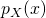, for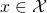.

Suppose we have a quantity of interest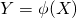.

We know that the function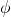is invertible, so eachmaps to exactly oneand vice versa.To help us remember the input/output of the transformation function, sometimes I’ll writeWe wish to find the density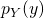of random variable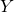, for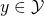.There are two pieces here on the RHS, which together create a density over the variable.

• the density function• Theterm takes a transformed version ofas input instead of. This determines the general shape of the resulting density.

• the Jacobian determinant• The determinant of the Jacobian of the transformation fromtocaptures how the change of variables “warps” the density function. For example, ifis a tiny fraction of, the Jacobian makes sure that a small change in the density ofis comparable to a large change in the original density.

For 1D variables, the Jacobian is simply the first-derivative:For multivariate, letdenote the-th dimension of the resulting vector. Then## Simple Multiplication in 1D

Consider the standard 1D Gaussian random variable:.Now, what is the distribution of?

First, we use simple algebra to identify the reverse transform:. So we’ll replacewith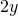in the density function.

Next, we compute the Jacobian term:.

Finally, we plug these results into the final density equation:when. So we recover the density for, as expected!

## 2D Gaussian Transformation from Cartesian to Polar

We start with a simple 2D zero-mean standard spherical Gaussian.and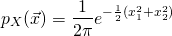We wish to transform to new variables, where the domain changes to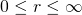and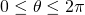.

Step 1: Find the inverse mapping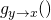. Standard results show this to be:Step 2: Compute the Jacobian matrix and find its determinant: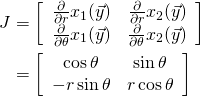Using the simple rule for 2×2 determinants, we find.

Finally, we come to Step 3: plug these results into the general formula.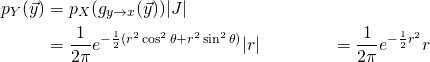where since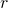is always positive,.

Importantly, keep in mind that this density is a function of bothand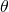, even thoughdoes not appear in the density function. This implies the distribution is uniform over all possible angles, which makes sense because the Gaussian is spherically symmetric.

## Aside: Calculating the normalization constant of the Gaussian

We can use the polar form of the 2D Gaussian to figure out why the peskynormalization constant always appears in every Gaussian PDF formula.

We can show using high school calculus thatis the normalization constant for the generic density shape given by.

Integrating over bothand, we find`uUsing the substitution, which enforcesbut leaves the bounds the same, we haveThus, the normalization constant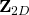for the 2D standard Gaussian is just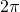.

Finally, since the 2D spherical Gaussian is the product of two independent 1D Gaussians, the normalization constant for the 1D case must be simply \$\mathbf{Z}_{1D} = \sqrt(2\pi) = \int_{-\infty}^{\infty} \
e.